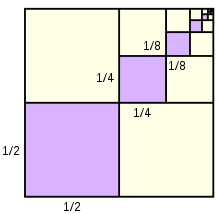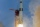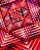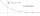# Arithmetic progression 2

The 3rd term of an Arithmetic progression is ten more than the first term, while the fifth term is 15 more than the second term. Find the sum of the 8th and 15th terms of the Arithmetic progression if the 7th term is seven times the first term.

s1 =  115

### Step-by-step explanation:Did you find an error or inaccuracy? Feel free to write us. Thank you!Tips to related online calculators
Do you have a linear equation or system of equations and looking for its solution? Or do you have a quadratic equation?

## Related math problems and questions:

• Common differenceThe 4th term of an arithmetic progression is 6. If the sum of the 8th and 9th terms is -72, find the common difference.
• Geometric progressiobIf the sum of four consective terms of geometric progression is 80 and arithmetic mean of second and fourth term is 30 then find terms?
• Fifth memberDetermine the fifth member of the arithmetic progression, if the sum of the second and fifth members equal to 73, and difference d = 7.
• 15th term of APWhat is the 15th term; x1=1.5, d=4.5?
• Missing term 2What is the missing term for the Geometric Progression (GP) 3, 15, 75,__, 1875?
• Sum 1-6Find the sum of the geometric progression 3, 15, 75,… to six terms.
• GP - three membersThe second and third of a geometric progression are 24 and 12(c+1), respectively, given that the sum of the first three terms of progression is 76. determine the value of c.
• Five elementThe geometric sequence is given by quotient q = 1/2 and the sum of the first six members S6 = 63. Find the fifth element a5.
• What is 10What is the 5th term, if the 8th term is 80 and common ratio r =1/2?
• Six termsFind the first six terms of the sequence a1 = -3, an = 2 * an-1
• HP - harmonic progressionDetermine the 8th term of the harmonic progression 2, 4/3, 1,…
• Geometric progressionIn geometric progression, a1 = 7, q = 5. Find the condition for n to sum first n members is sn≤217.
• HP - harmonic progression 2Compute the 16th term of the HP if the 6th and 11th term of the harmonic progression are 10 and 18 respectively.
• Trapezoid 15Area of trapezoid is 266. What value is x if bases b1 is 2x-3, b2 is 2x+1 and height h is x+4
• Sum of GP membersDetermine the sum of the GP 30, 6, 1.2, to 5 terms. What is the sum of all terms (to infinity)?
• RavensOn two trees sitting 17 ravens. If 3 ravens flew from first to second tree and 5 ravens took off from second tree then the first tree has 2 times more ravens than second tree. How many ravens was originally on every tree?
• If the 3If the 6th term of a GP is 4 and the 10th is 4/81, find common ratio r.